# Ribbon on the cube

A cubical gift box is tied with a piece of ribbon. If the total length of the free ends and the bow is 18 inches, what is the length of the ribbon used? (Each side of the cube is 6 inches).

x =  66 inch

### Step-by-step explanation: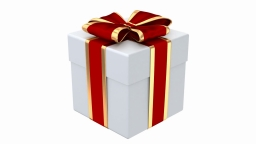Did you find an error or inaccuracy? Feel free to write us. Thank you!#### You need to know the following knowledge to solve this word math problem:

We encourage you to watch this tutorial video on this math problem:

## Related math problems and questions: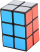A gift box has dimensions of: 8 1/2 inches, 5 1/2 inches, and 2 1/2 inches, respectively. How many cubes with side lengths of 1/2 inches would be needed to fill the gift box?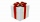How much wrapping paper is needed to wrap a cube-shaped gift with edge measuring 15 cm?
• PresentGift box has a rectangular shape with dimensions of 8×8×3 cm. Miloslav wants to cover with square paper with sides of 18 cm. How much paper left him?
• A piece 2A piece of material measures 38.25 inches. Courtney cuts the piece of material into two pieces. One piece measures 19.5 inches. Which addition equation could be used to find the length m of the other piece of material?
• Two ribbonsThe total length of the two ribbons is 13 meters. If one ribbon is 7 and 5/8 meters long, what is the length of the other ribbon?
• How many 15How many six-eights are there in 18? Which of the following would you choose to solve this question? 18 x 6/8 or 18 : 6/8
• Jewelry box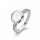The bottom of Zeyda's jewelry box is a rectangle with the length of 5 3/8 inches and a width of 3 1/4 inches. What is the area of the bottom of the jewelry box?
• KrishnaKrishna bought 5 1/2 meters of ribbon from the market . She cut off a 2 3/4 m piece of ribbon from it and gave it to Sushima . What length of ribbon is left with her?
• What is 8What is the perimeter of a rectangle with side lengths of ¾ inch and ⅔?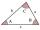The size of two internal angles of a triangle ABC are α=6/18π and β=7/18π. Calculate the size of the third angle.
• A pieceA piece of wire is 5.8 m long is to cut into 15 pieces of the same length what is the length of each piece?
• Into boxHow many cubes with an edge of 2.5 cm fit into a box measuring 11.6 cm; 8.9 cm and 13.75 cm?
• Triangles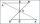Hanka cut the 20 cm long straws into three pieces each piece had a length in cm. Then, with these three pieces, she tried to make a triangle. a) What circuit has each of the triangles? b) How long can the longest side measure? c) How many different triang
• Sheet of paperIs the sheet of paper measuring 18 and 25 cm enough for taping 9 cm cubes with an edge? (1 = yes, 0 = no)
• Each ofEach of Suzan's notebooks is 4/5  inches wide. If she has 25 inches of space remaining on her bookshelf, how many notebooks will fit?
• Wax candles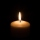A company makes wax candles in the shape of a cylinder. Each candle has a diameter of 4 inches and a height of 7 inches. If the company used 7561.12 in3 of wax, how many candles did it make? Use 3.14, and do not round your answer
• BoxCalculate the angle between box base 9 x 14 and body diagonal length 18.